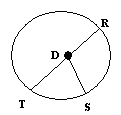Name: ___________________Date:___________________

 Email us to get an instant 20% discount on highly effective K-12 Math & English kwizNET Programs!

### Grade 4 - Mathematics4.5 Circle Facts - 2

 Circles are simple closed curves which divide the plane into an interior and exterior. Circle is a closed figure and not a polygon. A circle can be drawn with the help of a compass. All the points on a circle are at the same distance from the center of the circle. A line from the center to any point on the circle is called radius. Diameter is twice the radius. The circumference of a circle means the length of the circle. Directions: Answer the following. Draw a circle with compass and write all its properties.Name: ___________________Date:___________________

### Grade 4 - Mathematics4.5 Circle Facts - 2

 Q 1: Circle is a closed figure and is not a ________quadrilateralsquarepolygon Q 2: All the points on a circle are at the same distance from thepoints on the circlecenter of the circlepoints outside the circle Q 3: In the circle shown what the segments of equal lengthDR, DR and DSDT, TR and DSDT, DR and DS Q 4: A line segment whose end points are the center of the circle and point on the circle is calledlinediameterradiusline segment Q 5: All the radii of a circle have thesame lengthsdifferent lengths Q 6: The diameter of a circle ishalf the radiussame as radiustwice the radius Question 7: This question is available to subscribers only! Question 8: This question is available to subscribers only!